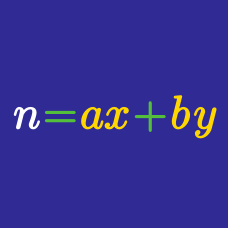Number Theory

System of Linear Diophantine Equations

If we solve the following system of equations $x + y + 2z = 10 \\ 3x - y + z = 5,$ where $x, y, z$ are non-negative integers, what is the sum of all possible values of $x?$

In the post office they sell souvenir stamps of three denominations: 25 cents, 10 cents, and 5 cents. If you want to buy 20 stamps for exactly 2 dollars, how many combinations of the three kinds of stamps are there that you can buy?

NOTE: It's possible, in a combination, to buy zero of a stamp variety.

Old Spinner's Manufacture employed 20 people at their shop. Their weekly salary was 3 shillings for a man, 2 shillings for a woman, and half a shilling for a child below 15. If the 20 people altogether received exactly 20 shillings a week and there were at least one man and one woman, how many children were employed?

Solve the following system of linear Diophantine equations $\left \{ \begin{array}{ll} 3x -4y -z = 5 \qquad & (1) \\ 3y -4z +2w = -5 \qquad & (2) \\ 2x + w = 10 \qquad & (3) \end{array} \right.$ for non-negative integers $x, y, z, w.$ Find the value of $x+ y+ z + w.$

Jane bought 5 watermelons, 3 apples and 20 cherries for 46 dollars. Lizzy bought 2 watermelons, 5 apples and 12 cherries for 27 dollars. If the prices of a watermelon and an apple are both integers, what is the price of an apple?